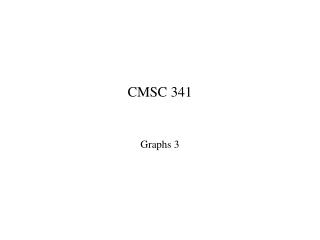DownloadDownload PresentationCMSC 341

# CMSC 341

Télécharger la présentation## CMSC 341

- - - - - - - - - - - - - - - - - - - - - - - - - - - E N D - - - - - - - - - - - - - - - - - - - - - - - - - - -
##### Presentation Transcript

1. CMSC 341 Graphs 3

2. Adjacency Table Implementation • Uses table of size |V|  |V| where each entry (i,j) is boolean • TRUE if there is an edge from vertex i to vertex j • FALSE otherwise • store weights when edges are weighted a a b e b e c d c d

3. Adjacency Table (cont.) • Storage requirement: • Performance:

4. Adjacency List • Keep list of adjacent vertices for each vertex. • Array of lists of indices: Each element of array[i] is a list of the indices of the vertices adjacent to vertex i. • List of lists: The i-th element of L is associated with vertex vi and is a List Li of the elements of L adjacent to vi. • Lists in Array (NIL sentinels): Each entry a[i,j] is either the index of the j-th vertex adjacent to vertex I or a NIL sentinel indicating end-of-list. • Lists in Array (with valence array): Instead of using NIL sentinels to mark the end of the list in the array, a separate array Valence is kept indicating the number of entries in each row of the array.

5. Adjacency Lists (cont.) • Storage requirement: • Performance: Array of List of Lists in Lists in • Lists Lists Array (NIL) Array (val)

6. Directed Acyclic Graphs • A directed acyclic graph is a directed graph with no cycles. • A partial order R on a set S is a binary relation such that • for all aS, aRa is false (irreflexive property) • for all a,b,c S, if aRb and bRc then aRc is true (transitive property) • To represent a partial order with a DAG: • represent each member of S as a vertex • for each pair of vertices (a,b), insert an edge from a to b if and only if aRb

7. More Definitions • Vertex i is a predecessor of vertex j if and only if there is a path from i to j. • Vertex i is an immediate predecessor if vertex j if and only if (i,j) is an edge in the graph. • Vertex j is a successor of vertex i if and only if there is a path from i to j. • Vertex j is an immediate predecessor if vertex i if and only if (i,j) is an edge in the graph.

8. Topological Ordering • A topological ordering of the vertices of a DAG G=(V,E) is a linear ordering such that, for vertices i,j V, if i is a predecessor of j, then i precedes j in the linear order.

9. Topological Sort • void TopSort(Graph G) { • unsigned int counter = 1 ; • Queue q = new Queue(); • Vertex indegree[|V|]; • for each Vertex v { • indegree[v] = getInDegree(v); • if (indegree[v] == 0) q.enqueue(v); } • while (!q.isEmpty()){ • v = q.dequeue(); • Put v on the topological ordering; • counter++; • for each Vertex v adjacent from v { • indegree[w] -=1; • if (indegree[w]==0) q.enqueue(w); • } • } • if (counter <= G.numVertices()) • declare an error -- G has a cycle • }

10. TopSort Example • 1 2 3 4 5 • 6 7 8 9 10